# SAT II Math I : Finding Angles with Trigonometry

## Example Questions

### Example Question #2 : Trigonometry

What is the measure of the angle made between a line segment with points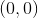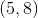and the-axis?  Round your answer to the nearest hundreth of a degree.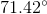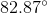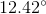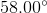No angle measure can be calculatedExplanation:

Based on the information given, we know that the ratio oftoon this segment could be represented as: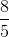This ratio represents the tangent of the triangle formed by our line segment and the-axis.  Using the inverse tangent function, we can find the angle measure: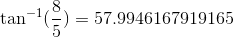This refers to a reference angle of### Example Question #2 : Trigonometry

What is the measure of the angle made between a line segment with points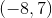and the-axis? Round your answer to the nearest hundreth of a degree.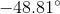No angle can be calculated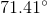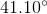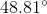Explanation:

Based on the information given, we know that the ratio oftoon this segment could be represented as: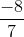This ratio represents the tangent of the triangle formed by our line segment and the-axis. Using the inverse tangent function, we can find the angle measure: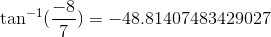This refers to a reference angle of.

### Example Question #7 : Trigonometry

A triangle is formed by connecting the points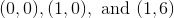.  Determine the elevation angle to the nearest integer in degrees.Explanation:

After connecting the points on the graph, the length of the triangular base is 1 unit.

The height of the triangle is 6.  To find the elevation angle, the angle is opposite from the height of the triangle.  Since we know the base and the height, the elevation angle can be solved by using the property of tangent.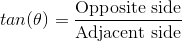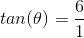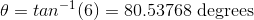The best answer is.